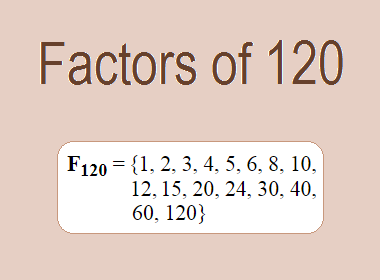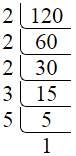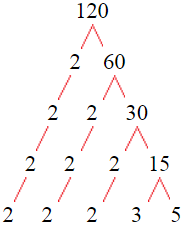# Factors of 120The factors of 120 are 1, 2, 3, 4, 5, 6, 8, 10, 12, 15, 20, 24, 30, 40, 60, and 120 i.e. F120 = {1, 2, 3, 4, 5, 6, 8, 10, 12, 15, 20, 24, 30, 40, 60, 120}. The factors of 120 are all the numbers that can divide 120 without leaving a remainder.

We can check if these numbers are factors of 120 by dividing 120 by each of them. If the result is a whole number, then the number is a factor of 120. Let's do this for each of the numbers listed above:

·        1 is a factor of 120 because 120 divided by 1 is 120.

·        2 is a factor of 120 because 120 divided by 2 is 60.

·        3 is a factor of 120 because 120 divided by 3 is 40.

·        4 is a factor of 120 because 120 divided by 4 is 30.

·        5 is a factor of 120 because 120 divided by 5 is 24.

·        6 is a factor of 120 because 120 divided by 6 is 20.

·        8 is a factor of 120 because 120 divided by 8 is 15.

·        10 is a factor of 120 because 120 divided by 10 is 12.

·        12 is a factor of 120 because 120 divided by 12 is 10.

·        15 is a factor of 120 because 120 divided by 15 is 8.

·        20 is a factor of 120 because 120 divided by 20 is 6.

·        24 is a factor of 120 because 120 divided by 24 is 5.

·        30 is a factor of 120 because 120 divided by 30 is 4.

·        40 is a factor of 120 because 120 divided by 40 is 3.

·        60 is a factor of 120 because 120 divided by 60 is 2.

·        120 is a factor of 120 because 120 divided by 120 is 1.

## How to Find Factors of 120?

1 and the number itself are the factors of every number. So, 1 and 120 are two factors of 120. To find the other factors of 120, we can start by dividing 120 by the numbers between 1 and 120. If we divide 120 by 2, we get a remainder of 0. Therefore, 2 is a factor of 120. If we divide 120 by 3, we get a remainder of 0. Therefore, 3 is a factor of 120.

Next, we can check if 4 is a factor of 120. If we divide 120 by 4, we get a remainder of 0. Therefore, 4 is also a factor of 120. We can continue this process for all the possible factors of 120.

Through this process, we can find that the factors of 120 are 1, 2, 3, 4, 5, 6, 8, 10, 12, 15, 20, 24, 30, 40, 60, and 120. These are the only numbers that can divide 120 without leaving a remainder.

********************

********************

## Properties of the Factors of 120

The factors of 120 have some interesting properties. One of the properties is that the sum of the factors of 120 is equal to 360. We can see this by adding all the factors of 120 together:

1 + 2 + 3 + 4 + 5 + 6 + 8 + 10 + 12 + 15 + 20 + 24 + 30 + 40 + 60 + 120 = 360

Another property of the factors of 120 is that the prime factors of 120 are 2, 3, and 5 only.

## Applications of the Factors of 120

The factors of 120 have several applications in mathematics. One of the applications is in finding the highest common factor (HCF) of two or more numbers. The HCF is the largest factor that two or more numbers have in common. For example, to find the HCF of 120 and 84, we need to find the factors of both numbers and identify the largest factor they have in common. The factors of 120 are 1, 2, 3, 4, 5, 6, 8, 10, 12, 15, 20, 24, 30, 40, 60, and 120. The factors of 84 are 1, 2, 3, 4, 6, 7, 12, 14, 21, 28, 42, and 84. The largest factor that they have in common is 12. Therefore, the HCF of 120 and 84 is 12.

Another application of the factors of 120 is in prime factorization. Prime factorization is the process of expressing a number as the product of its prime factors. The prime factors of 120 are 2, 3, and 5, since these are the only prime numbers that can divide 120 without leaving a remainder. Therefore, we can express 120 as:

120 = 2 × 2 × 2 × 3 × 5

We can do prime factorization by division and factor tree method also. Here is the prime factorization of 120 by division method,120 = 2 × 2 × 2 × 3 × 5

Here is the prime factorization of 120 by the factor tree method,120 = 2 × 2 × 2 × 3 × 5

## Conclusion

The factors of 120 are the numbers that can divide 120 without leaving a remainder. The factors of 120 are 1, 2, 3, 4, 5, 6, 8, 10, 12, 15, 20, 24, 30, 40, 60, and 120. The factors of 120 have some interesting properties, such as having a sum of 360. The factors of 120 have several applications in mathematics, such as finding the highest common factor and prime factorization.